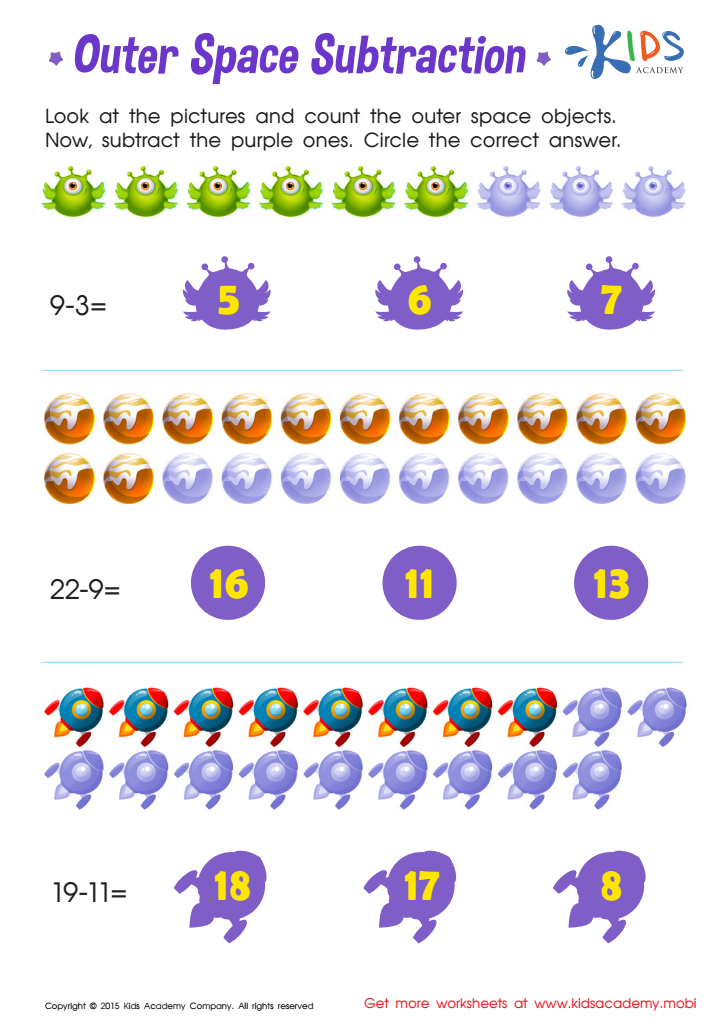8 filtered results

Clear all filters
Favorites
Online only

8 filtered results

Difficulty Level

Standards

Introducing our Easy Subtraction worksheets with answer key, designed specially for Grade 1 students. These worksheets are a fantastic resource to help young learners develop their subtraction skills effectively. With a variety of engaging exercises, children will be able to practice subtraction in a fun and interactive way. The answer key included allows for quick and easy checking, promoting independent learning. Our Easy Subtraction worksheets are a great addition to any classroom or homeschooling environment, encouraging students to build a strong foundation in mathematics. Order yours today and watch your students excel in subtraction!

Favorites
Online only
• Subtraction
• Easy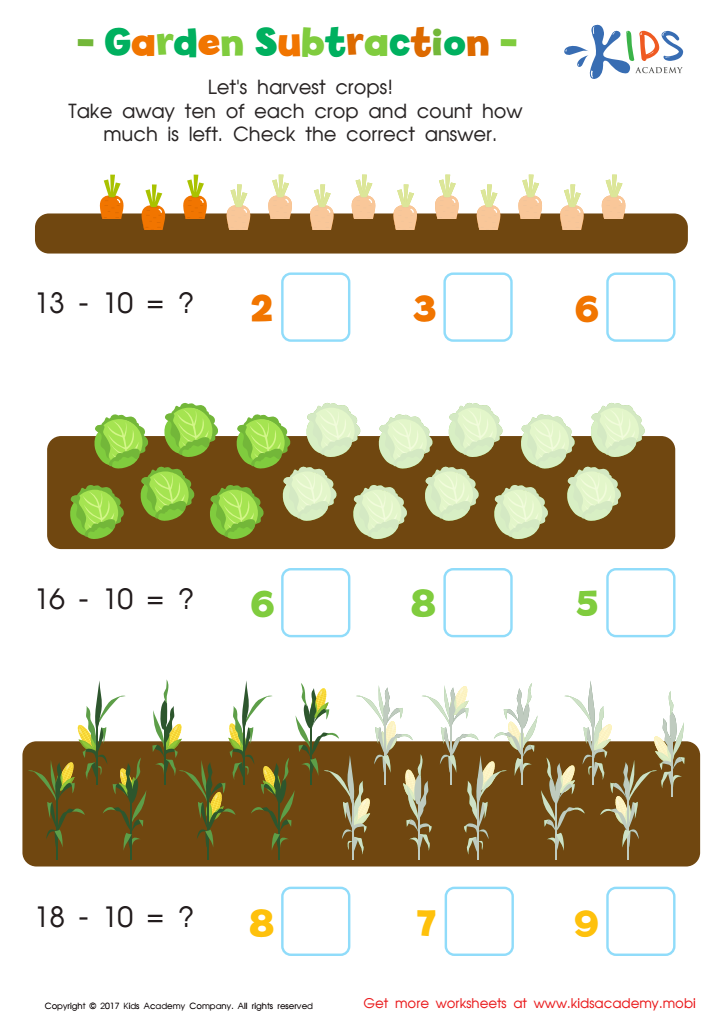Garden Subtraction Worksheet
Help your kids understand math with this fun, garden-themed subtraction worksheet! Have them read the number sentences and count the veggies in each row. Then, subtract 10 from each row to find the answer. They'll love counting the colorful veggies and solving the problems!
Garden Subtraction Worksheet
Worksheet
Garden Subtraction Worksheet
Number sentences are difficult to solve if students don’t understand the underlying concept behind the …
Subtraction
Worksheet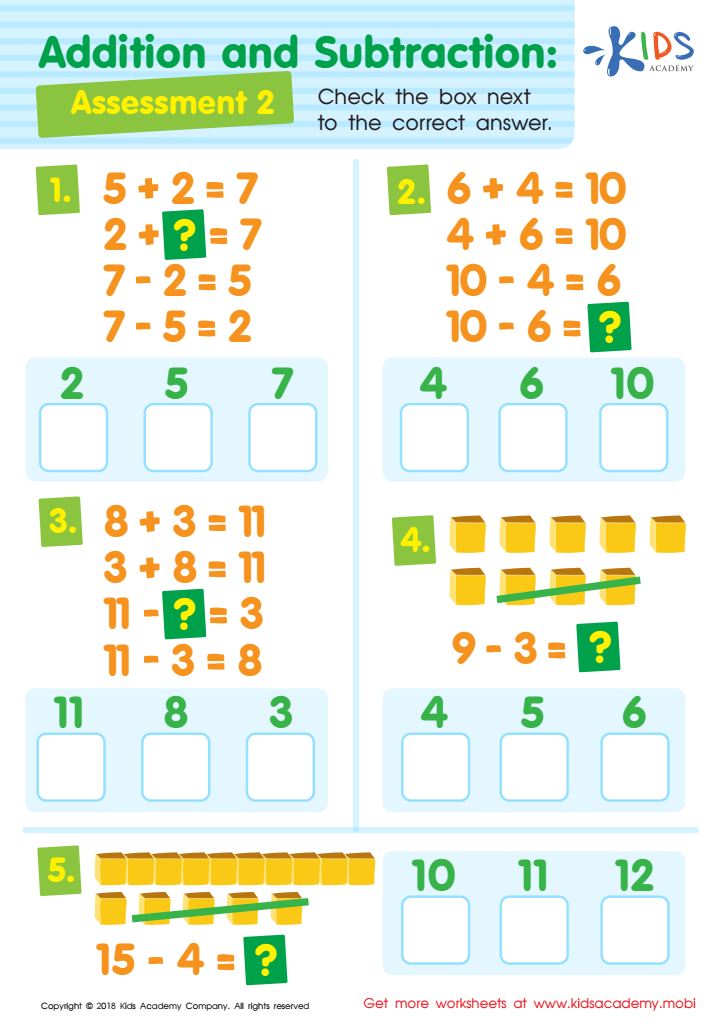Addition and Subtraction Assessment 2 Worksheet
As your child grows, math problems become harder. To keep their brains active and provide extra help if needed, use this math worksheet. It'll help them become more familiar with equations. Go through each one carefully with your child and have them check the box next to the right answer.
Addition and Subtraction Assessment 2 Worksheet
Worksheet
Addition and Subtraction Assessment 2 Worksheet
As your child progresses in age and class, the mathematical problems they have to solve …
Subtraction
Worksheet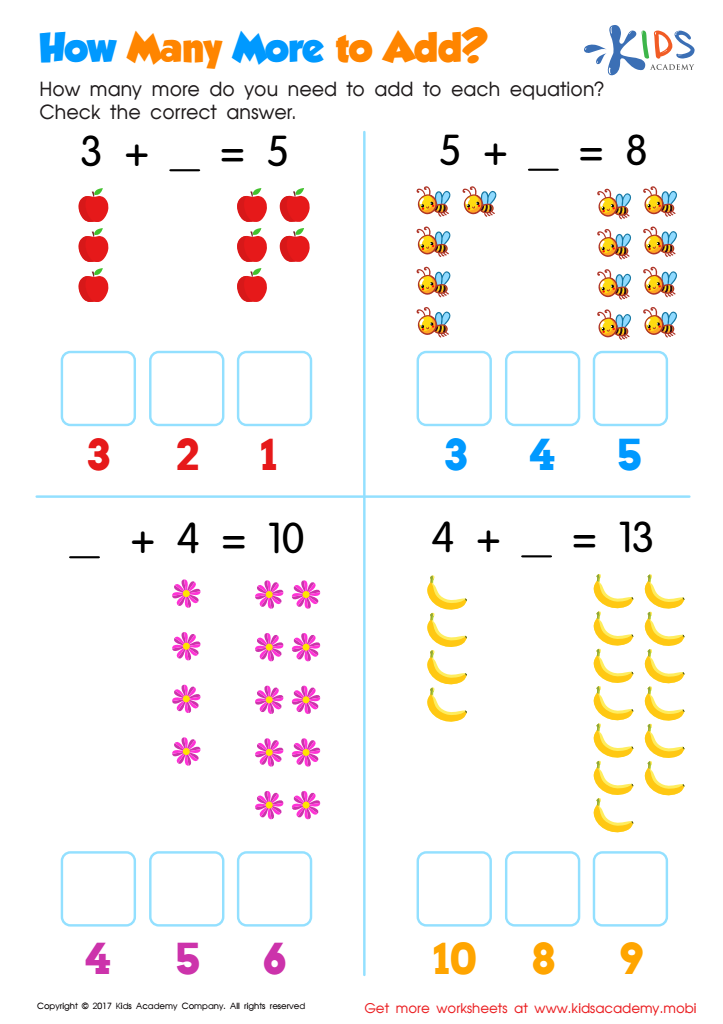How Many More to Add Worksheet
Sharpen your child's math skills with this pdf worksheet. Help them figure out how many apples, bees, flowers and bananas are needed to reach the answer given. The possible answers are provided at the bottom of the boxes. Have some fun doing some addition and subtraction magic together and get those math skills sharpened!
How Many More to Add Worksheet
Worksheet
How Many More to Add Worksheet
Sharpen your child’s addition and subtraction skills with the pdf worksheet. How many more apples, …
Subtraction
Worksheet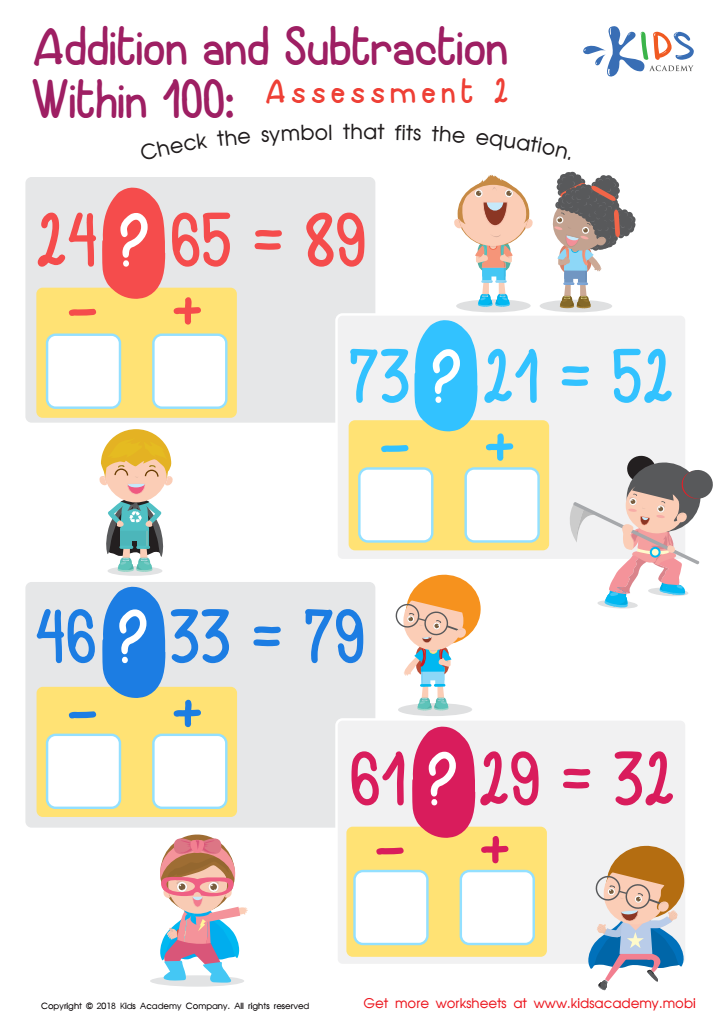Addition and Subtraction Within 1: Assessment 2 Worksheet
This worksheet is a great tool to help your students learn addition and subtraction. It contains four equations in a printable pdf. Ask your students to review the equation and determine if the symbol between them is a '+' or '-'. Guide them in selecting the correct symbols for each equation.
Addition and Subtraction Within 1: Assessment 2 Worksheet
Worksheet
Addition and Subtraction Within 1: Assessment 2 Worksheet
If you are teaching your students addition and subtraction in class now, this worksheet will …